Still have questions?
Contact support

# Technical Indicators Mathematical Description

## Overview

This document contains mathematical description of all technical indicators available in AnyChart Stock Component.

For all formulas and indicators below:

• n is a period for which calculations are done, it is usually set by the period parameter of the method that creates an indicator.
• Xi is the value passed by the data source.

## Accumulation Distribution Line

Accumulation Distribution Line is calculated according to these formulas: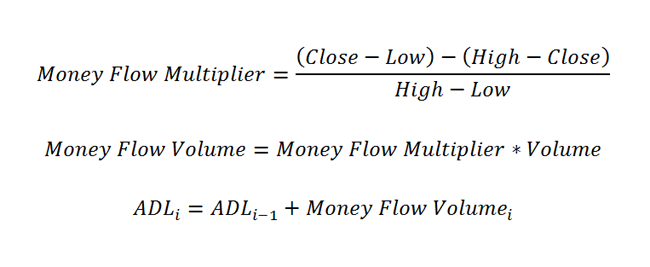Each point of the Adaptive Moving Average indicator is calculated by the following steps:

1. Calculate the ER:

2.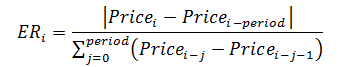3. Then, use the following formula to calculate the SSC value:

4.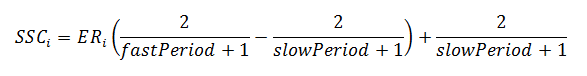5. Now, use the results from the actions made before and calculate the indicator:

6.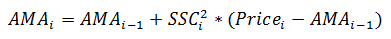## Aroon

Aroon indicator is calculated according to the following formula: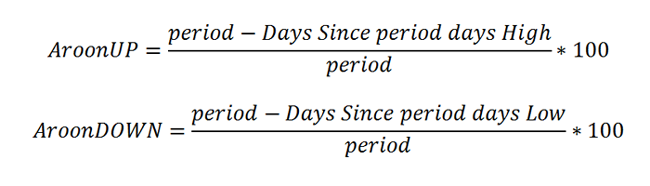## Average True Range

Average True Range in each point is calculated according to the following formula: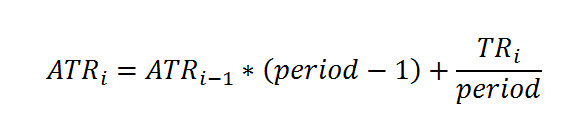This formula is based on the True Range definition. ATR is defined as the greatest of the following:

• current High less than current Low
• current High less than previous Close (absolute value)
• current Low less than previous Close (absolute value)

## Bollinger Bands

Bollinger Bands indicator is calculated in three steps:

1. Calculate the SMA according to the SMA formula.

2. Use the next formula to calculate the standard deviation:

3.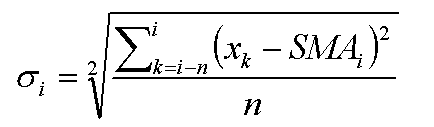4. Bollinger Bands values are calculated according to this formula, where "d" is a deviation:

5.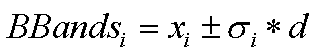## Bollinger Bands %B

The Bollinger Bands %B indicator is calculated according to the following formula: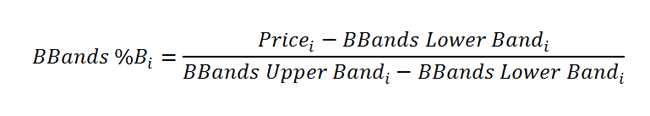## Bollinger Bands Width

The Bollinger Bands Width article indicator is calculated according to the following formula:

``````bandwidth = (upperBB − lowerBB) / middleBB
``````

## Commodity Channel Index

Commodity Channel Index is calculated according to this formula: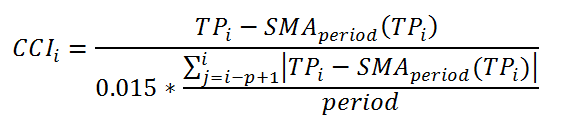Where TP is a Typical Price calculated as: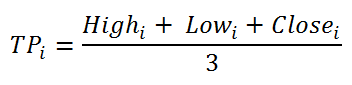And SMA is calculated as stated in SMA part of this article.

## Chaikin Money Flow

Chaikin Money Flow is calculated in three steps.

1. First a MFM(i) is calculated for all points according to this formula:

2.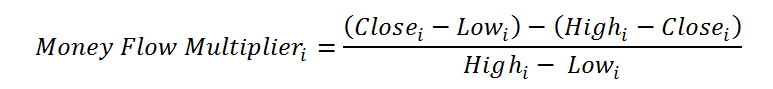3. Next an MFV(i) value is calculated for all points like this:

4.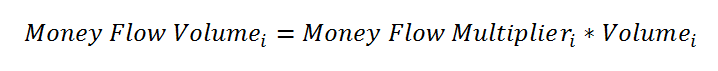5. And finally the CMF(i) value is calculated according to this formula:

6.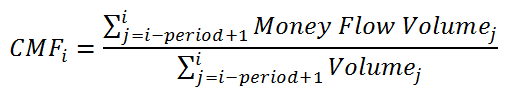## Chaikin Oscillator

Chaikin Oscillator is calculated according to this formula: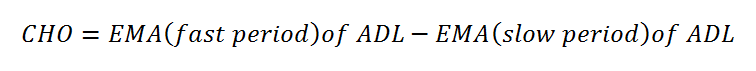## Chaikin Volatility

Coming soon.

Chaikin Volatility indicator in each point is calculated according to the following formula: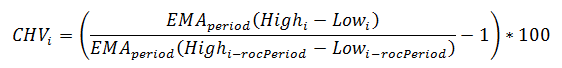## Directional Movement Indicator

Directional Movement Indicator is calculated in three steps:

1. First the True Range (TR), Plus Directional Movement (+DM) and Minus Directional Movement (-DM) are calculated for each period:

2.3. Next they are smoothed using EMA or Wilder's method, calculated as:

4.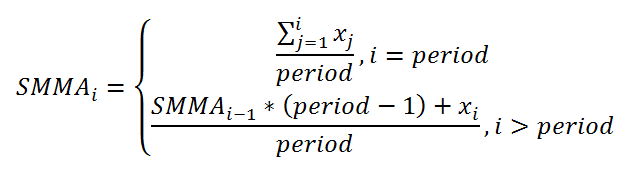5. And finally the +DI and -DI values are calculated according to these formulas:

6.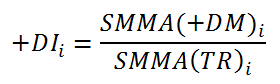## Exponential Moving Average

Exponential Moving Average in each point is calculated according to the following formula: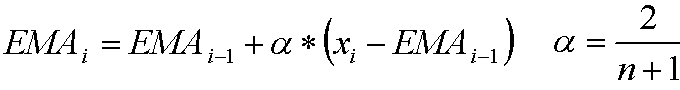## KDJ

KDJ is calculated quite alike Stochastic indicator, but the difference is in having a J line, which Stochastic does not have.

1. The %K line is calculated the following way:

2.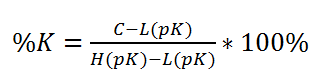3. To create the %D line, use the next formula:

4.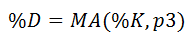5. This is how the %J line is calculated:

6.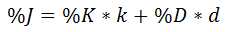Coming soon.

## Modified Moving Average

Modified Moving Average indicator points are calculated by the following formula: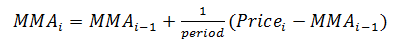Coming soon.

Coming soon.

## Moving Average Convergence Divergence

Moving Average Convergence Divergence indicator is calculated as follows:

1. EMA[slow period]i and EMA[fast period]i is calculated by EMA formula. Slow period and fast period are set by slowPeriod (default 26) and fastPeriod (default 12) parameters of macd() method.
2. MACD series value is calculated: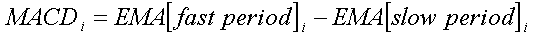3. EMA[signal period]i of MACD series values is calculated by EMA formula, where signal period is set by signalPeriod parameter.
4. Signal series value is calculated: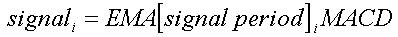5. Histogram series is calculated: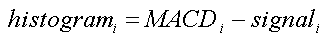Coming soon.

Coming soon.

Coming soon.

Coming soon.

## Rate of Change

Rate of Change is calculated according to this formula: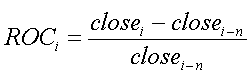## Relative Strength Index

Relative Strength Index is calculated as follows:

1. Upward change (U) or downward change (D) sequences are calculated: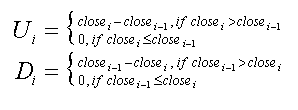2. Then two averages are calculated: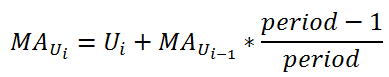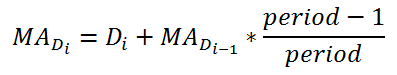3. And final RSI formula is :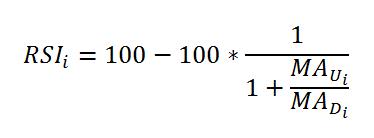## Simple Moving Average

Simple Moving Average in each point is calculated according to the following formula: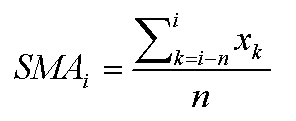## Stochastic Oscillator

Stochastic Oscillator indicator has two series, both being calculated with the help of other indicators (EMA or SMA). Also it has 3 types: Fast Stochastic Oscillator, Slow Stochastic Oscillator and Full Stochastic Oscillator.

The defaults create a Fast Stochastic Oscillator indicator.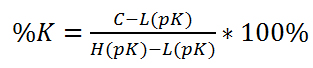where pK is the first period that is set through the stochastic() method, which is a period for the %K value.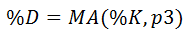where p3 is the third period that is set through the stochastic() method, which is a period for the %D value.

While Fast Stochastic Oscillator is used for signals, the Slow Stochastic Oscillator is supposed to reflect this emphasis.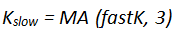where 3 is a default period for getting slow K.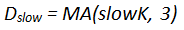where 3 is a default period for getting slow D.

The Full Stochastic Oscillator is a fully customizable version of the Slow Stochastic Oscillator. Users can set the look-back period, the number of periods to slow %K and the number of periods for the %D moving average.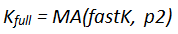where p2 is the second period that is set through the stochastic() method, which is a period for the smoothed %K value.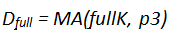where p3 is the third period that is set through the stochastic() method, which is a period for the %D value.

Coming soon.

Coming soon.

## Williams %R

Coming soon.

You are looking at an outdated v7 version of this document. Switch to the v8 version to see the up to date information.